I've been using an opaque wire frame helix as my profile image during my time on Brilliant. About ten years ago, I became interested in how to render and display images, particularly ones in which certain parts of an object block other parts of the image from view. Around that same time, I wrote some very primitive Python code to generate the opaque helix. At a high level, it works like this;

1) There are two basic elements to the image: points and surfaces
2) The points form the wire frame object (you can see the individual points if you look closely)
3) The surfaces are similar to rectangles, and each "rectangle" is divided into two triangles
4) To display the image, a line (or "ray") is traced from every point to an observer's location
5) The ray's path is checked for intersections with every single triangle
6) If the ray does not intersect any triangle, the corresponding point is displayed; otherwise, it is hidden

I have attached the Python code, as well as some images of the helix in different orientations. The exact functioning of the code is beyond the scope of this note, but it is possible to generate images of the helix in different orientations by running with different values of the angular parameters "disprotxy", "disprotxz", and "disprotyz" (near the top of the code).

The Python code spits out $(x,y)$ pairs, which can be visualized as a scatter plot in Microsoft Excel. Click the images below to enlarge them.

I'm somewhat proud to say that the code does not call any fancy libraries of image processing routines. It just uses elementary math functions, loops, and arrays.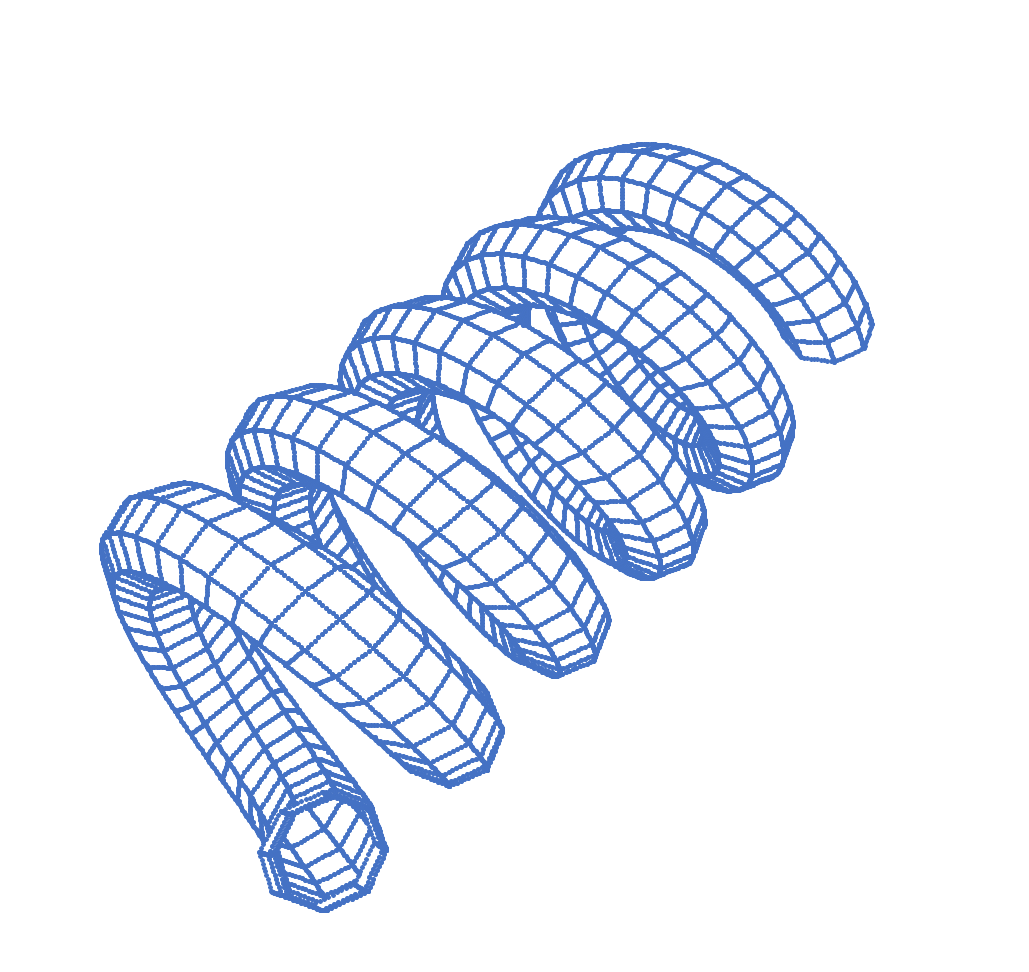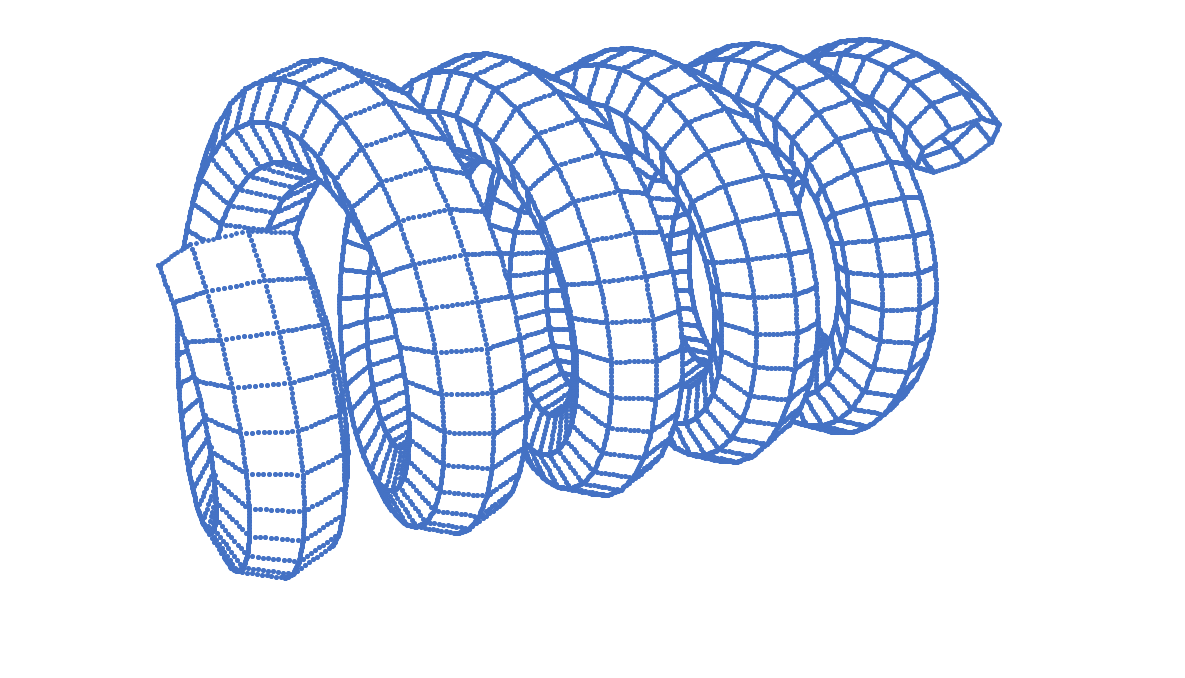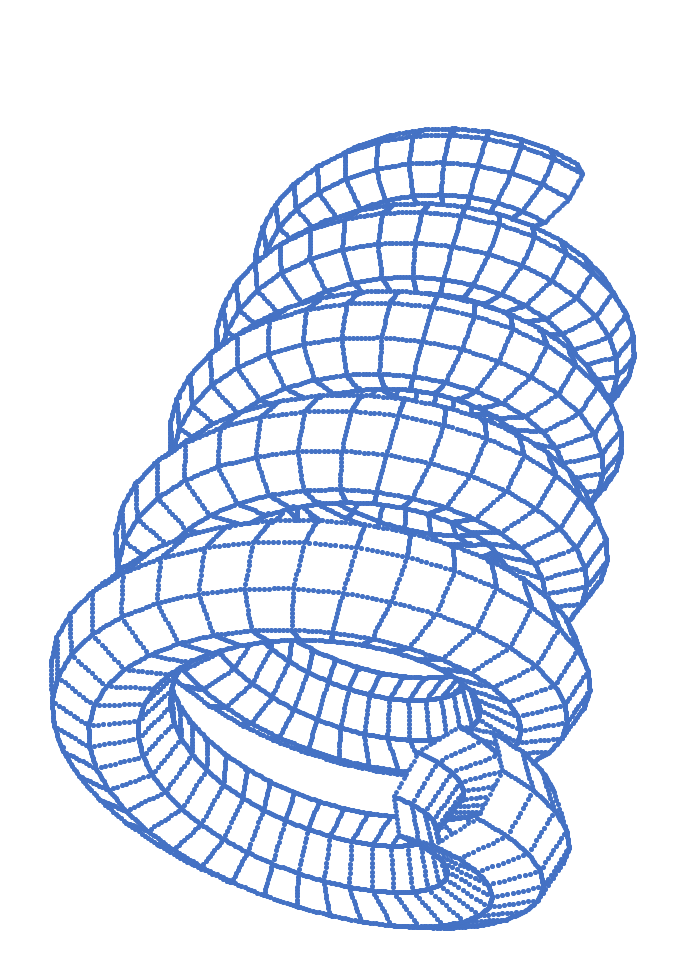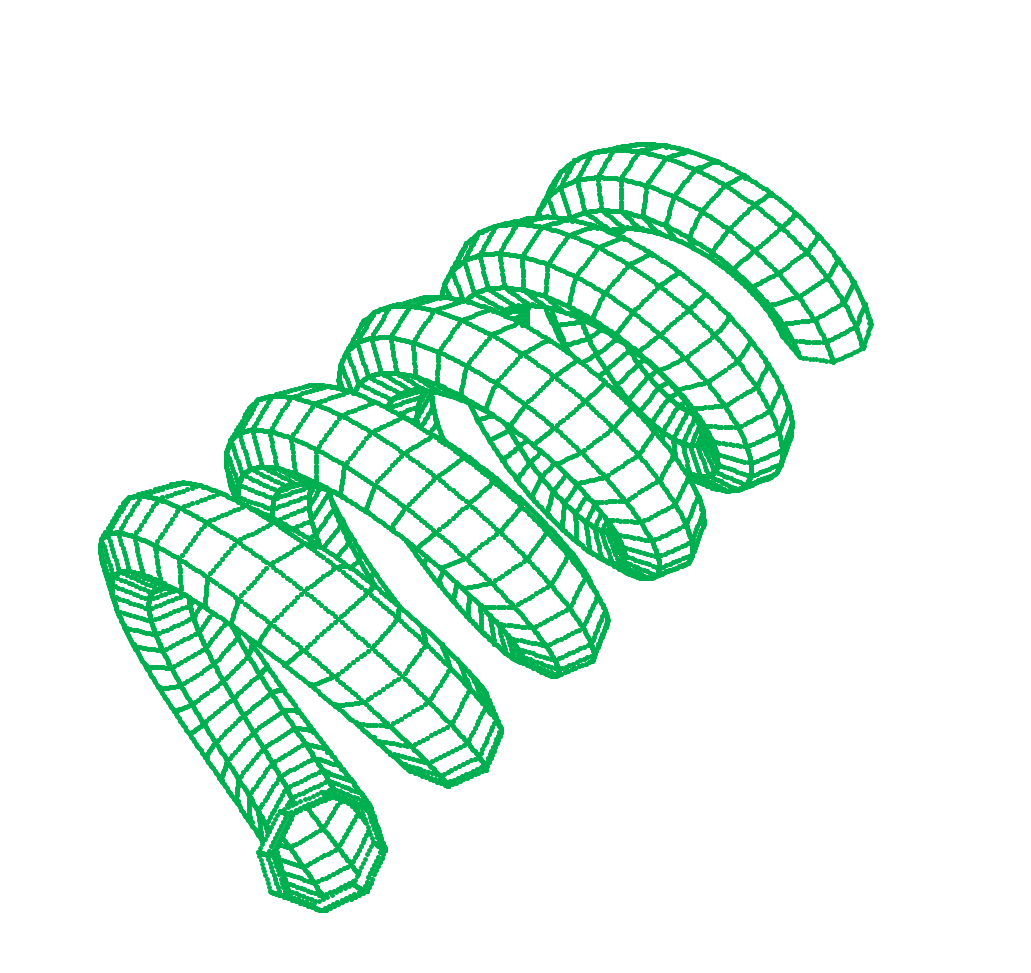1 2 3 4 5 6 7 8 9 10 11 12 13 14 15 16 17 18 19 20 21 22 23 24 25 26 27 28 29 30 31 32 33 34 35 36 37 38 39 40 41 42 43 44 45 46 47 48 49 50 51 52 53 54 55 56 57 58 59 60 61 62 63 64 65 66 67 68 69 70 71 72 73 74 75 76 77 78 79 80 81 82 83 84 85 86 87 88 89 90 91 92 93 94 95 96 97 98 99 100 101 102 103 104 105 106 107 108 109 110 111 112 113 114 115 116 117 118 119 120 121 122 123 124 125 126 127 128 129 130 131 132 133 134 135 136 137 138 139 140 141 142 143 144 145 146 147 148 149 150 151 152 153 154 155 156 157 158 159 160 161 162 163 164 165 166 167 168 169 170 171 172 173 174 175 176 177 178 179 180 181 182 183 184 185 186 187 188 189 190 191 192 193 194 195 196 197 198 199 200 201 202 203 204 205 206 207 208 209 210 211 212 213 214 215 216 217 218 219 220 221 222 223 224 225 226 227 228 229 230 231 232 233 234 235 236 237 238 239 240 241 242 243 244 245 246 247 248 249 250 251 252 253 254 255 256 257 258 259 260 261 262 263 264 265 266 267 268 269 270 271 272 273 274 275 276 277 278 279 280 281 282 283 284 285 286 287 288 289 290 291 292 293 294 295 296 297 298 299 300 301 302 303 304 305 306 307 308 309 310 311 312 313 314 315 316 317 318 319 320 321 322 323 324 325 326 327 328 329 330 331 332 333 334 import math import copy numrev = 5 # Number of revolutions numper = 36 # Number of frames per revolution Rt = 1.0 # Toroid radius Rcs = .3 # Toroid cross section Nf = numrev * numper # Number of cross section frames Ns = 8 # Number of sides for the cross section thetat = 0.0 # Angle in the xy plane at which a cross sectional frame is located (toroid originally lies in the xy plane with z axis as the central axis) dthetat = 2.0 * math.pi / numper # xy plane angular separation between cross sectional frames thetacs = 0.0 # Angle for determinng cross section points dthetacs = 2.0 * math.pi / Ns # Angular separation between the points in a cross section disprotxy = (10.0 / 180.0) * math.pi # Rotation angle in the xy plane for display #10 is default disprotxz = (40.0 / 180.0) * math.pi # Rotation angle in the xz plane for display # 40 is default disprotyz = (50.0 / 180.0) * math.pi # Rotation angle in the yz plane for display # 50 is default coord = [] # xyz coordinates of a point within the toroid / frame frame = [] # Array containing coordinates for all points within a frame toroid = [] # Array containing all frames within the toroid points = [] # Master array of all points block = [] # Blocking array # Create the frames # Initialize xyz coordinates x = 0.0 y = 0.0 z = 0.0 for k in range(0,Nf): # For each frame frame = [] for j in range(0,Ns): # Assign coordinates to every point within the frame and append to the frame array coord = [] x = (Rt + Rcs * math.cos(thetacs))*math.cos(k*dthetat) y = (Rt + Rcs * math.cos(thetacs))*math.sin(k*dthetat) z = Rcs * math.sin(thetacs) + k * (3.0 * Rcs)/(numper) coord.append(x) coord.append(y) coord.append(z) frame.append(coord[:]) thetacs = thetacs + dthetacs toroid.append(frame[:]) # Append first frame to the toroid array # Apply display rotations to all points within the toroid x = 0.0 y = 0.0 z = 0.0 Rxy = 0.0 thetaxy = 0.0 Rxz = 0.0 thetaxz = 0.0 Ryz = 0.0 thetayz = 0.0 for j in range(0,Nf): # For every frame for k in range(0,Ns): # For every point x = toroid[j][k] y = toroid[j][k] z = toroid[j][k] Rxy = math.hypot(x,y) thetaxy = math.atan2(y,x) x = Rxy * math.cos(thetaxy + disprotxy) y = Rxy * math.sin(thetaxy + disprotxy) Rxz = math.hypot(x,z) thetaxz = math.atan2(z,x) x = Rxz * math.cos(thetaxz + disprotxz) z = Rxz * math.sin(thetaxz + disprotxz) Ryz = math.hypot(z,y) thetayz = math.atan2(z,y) y = Ryz * math.cos(thetayz + disprotyz) z = Ryz * math.sin(thetayz + disprotyz) toroid[j][k] = x toroid[j][k] = y toroid[j][k] = z #print toroid[j][k],toroid[j][k],toroid[j][k] # Form master point array for j in range(0,Nf-1): # For every frame for k in range(0,Ns): # For every point xp = toroid[j][k] yp = toroid[j][k] zp = toroid[j][k] diffx = (toroid[j][k-1] - xp) / 10.0 diffy = (toroid[j][k-1] - yp) / 10.0 diffz = (toroid[j][k-1] - zp) / 10.0 for m in range(0,10): points.append([xp,yp,zp]) xp = xp + diffx yp = yp + diffy zp = zp + diffz diffx = (toroid[j+1][k-1] - xp) / 10.0 diffy = (toroid[j+1][k-1] - yp) / 10.0 diffz = (toroid[j+1][k-1] - zp) / 10.0 for m in range(0,10): points.append([xp,yp,zp]) xp = xp + diffx yp = yp + diffy zp = zp + diffz diffx = (toroid[j+1][k] - xp) / 10.0 diffy = (toroid[j+1][k] - yp) / 10.0 diffz = (toroid[j+1][k] - zp) / 10.0 for m in range(0,10): points.append([xp,yp,zp]) xp = xp + diffx yp = yp + diffy zp = zp + diffz diffx = (toroid[j][k] - xp) / 10.0 diffy = (toroid[j][k] - yp) / 10.0 diffz = (toroid[j][k] - zp) / 10.0 for m in range(0,10): points.append([xp,yp,zp]) xp = xp + diffx yp = yp + diffy zp = zp + diffz #for j in range(0,len(points)): #print points[j],points[j] # Form blocking surfaces for j in range(0,Nf-1): # For every frame for k in range(0,Ns): # For every cross section point block.append([[toroid[j][k],toroid[j][k],toroid[j][k]],[toroid[j][k-1],toroid[j][k-1],toroid[j][k-1]],[toroid[j+1][k],toroid[j+1][k],toroid[j+1][k]]]) block.append([[toroid[j+1][k-1],toroid[j+1][k-1],toroid[j+1][k-1]],[toroid[j][k-1],toroid[j][k-1],toroid[j][k-1]],[toroid[j+1][k],toroid[j+1][k],toroid[j+1][k]]]) Robs = 10.0 thetaobs = 0.0 phiobs = 0.0 screendist = 9.0 scsize = 1.0 screen = [[screendist,0.0,0.0],[math.hypot(scsize,screendist),math.atan2(scsize,screendist),0.0],[math.hypot(scsize,screendist),0.0,math.atan2(scsize,screendist)]] nn = 50.0 dtheta = (nn * 7.2 / 180.0) * math.pi dphi = (0.0 / 180.0) * math.pi thetaobs = thetaobs + dtheta screen = screen + dtheta screen = screen + dtheta screen = screen + dtheta phiobs = phiobs + dphi screen = screen + dphi screen = screen + dphi screen = screen + dphi Ox = Robs * math.sin(thetaobs) * math.cos(phiobs) Oy = Robs * math.sin(phiobs) Oz = Robs * math.cos(thetaobs) * math.cos(phiobs) dummy0 = screen[:] dummy1 = screen[:] dummy2 = screen[:] screen = dummy0 * math.sin(dummy0) * math.cos(dummy0) screen = dummy0 * math.sin(dummy0) screen = dummy0 * math.cos(dummy0) * math.cos(dummy0) screen = dummy1 * math.sin(dummy1) * math.cos(dummy1) screen = dummy1 * math.sin(dummy1) screen = dummy1 * math.cos(dummy1) * math.cos(dummy1) screen = dummy2 * math.sin(dummy2) * math.cos(dummy2) screen = dummy2 * math.sin(dummy2) screen = dummy2 * math.cos(dummy2) * math.cos(dummy2) #print Ox,Oy,Oz #print screen for j in range(0,len(points)): # For every point disp = 1 for m in range(0,len(block)): # Compare every point against each surface to find intersections B1x = (block[m] - block[m]) B1y = (block[m] - block[m]) B1z = (block[m] - block[m]) B2x = (block[m] - block[m]) B2y = (block[m] - block[m]) B2z = (block[m] - block[m]) Nx = B1y * B2z - B2y * B1z Ny = B2x * B1z - B1x * B2z Nz = B1x * B2y - B2x * B1y Px = points[j] Py = points[j] Pz = points[j] Cx = block[m] Cy = block[m] Cz = block[m] alpha = (Nx * (Cx - Px) + Ny * (Cy - Py) + Nz * (Cz - Pz)) / (Nx * (Ox - Px) + Ny * (Oy - Py) + Nz * (Oz - Pz)) if (alpha > 0.0) and (alpha <=1.0): # if the ray intersects the infinte planar region between P and O Rx = Px + alpha * (Ox - Px) Ry = Py + alpha * (Oy - Py) Rz = Pz + alpha * (Oz - Pz) vdeltax = Rx - Cx vdeltay = Ry - Cy vdeltaz = Rz - Cz B1x = B1x + .000000001 B2y = B2y + .000000001 sigma = (vdeltay - (B1y * vdeltax) / B1x) / (B2y - (B1y * B2x) / B1x) gamma = (vdeltax - sigma * B2x) / B1x if (sigma > 0.0) and (gamma > 0.0) and (sigma + gamma < 1) and (alpha > .007): # if the ray intersects a surface, don't display disp = 0 #print j,m if disp == 1: B1x = (screen - screen) / scsize B1y = (screen - screen) / scsize B1z = (screen - screen) / scsize B2x = (screen - screen) / scsize B2y = (screen - screen) / scsize B2z = (screen - screen) / scsize #print B1x,B1y,B1z,B2x,B2y,B2z Nx = B1y * B2z - B2y * B1z Ny = B2x * B1z - B1x * B2z Nz = B1x * B2y - B2x * B1y #print Nx,Ny,Nz Px = points[j] Py = points[j] Pz = points[j] Cx = screen Cy = screen Cz = screen #print Cx,Cy,Cz alpha = (Nx * (Cx - Px) + Ny * (Cy - Py) + Nz * (Cz - Pz)) / (Nx * (Ox - Px) + Ny * (Oy - Py) + Nz * (Oz - Pz)) #print alpha Rx = Px + alpha * (Ox - Px) Ry = Py + alpha * (Oy - Py) Rz = Pz + alpha * (Oz - Pz) vdeltax = Rx - Cx vdeltay = Ry - Cy vdeltaz = Rz - Cz #print vdeltax,vdeltay,vdeltaz B1x = B1x + .000000001 B2y = B2y + .000000001 sigma = (vdeltay - (B1y * vdeltax) / B1x) / (B2y - (B1y * B2x) / B1x) gamma = (vdeltax - sigma * B2x) / B1x if math.fabs(sigma) < scsize and math.fabs(gamma) < scsize: print gamma,sigma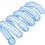Note by Steven Chase
1 year, 3 months ago

This discussion board is a place to discuss our Daily Challenges and the math and science related to those challenges. Explanations are more than just a solution — they should explain the steps and thinking strategies that you used to obtain the solution. Comments should further the discussion of math and science.

When posting on Brilliant:

• Use the emojis to react to an explanation, whether you're congratulating a job well done , or just really confused .
• Ask specific questions about the challenge or the steps in somebody's explanation. Well-posed questions can add a lot to the discussion, but posting "I don't understand!" doesn't help anyone.
• Try to contribute something new to the discussion, whether it is an extension, generalization or other idea related to the challenge.

MarkdownAppears as
*italics* or _italics_ italics
**bold** or __bold__ bold
- bulleted- list
• bulleted
• list
1. numbered2. list
1. numbered
2. list
Note: you must add a full line of space before and after lists for them to show up correctly
paragraph 1paragraph 2

paragraph 1

paragraph 2

[example link](https://brilliant.org)example link
> This is a quote
This is a quote
    # I indented these lines
# 4 spaces, and now they show
# up as a code block.

print "hello world"
# I indented these lines
# 4 spaces, and now they show
# up as a code block.

print "hello world"
MathAppears as
Remember to wrap math in $$ ... $$ or $ ... $ to ensure proper formatting.
2 \times 3 $2 \times 3$
2^{34} $2^{34}$
a_{i-1} $a_{i-1}$
\frac{2}{3} $\frac{2}{3}$
\sqrt{2} $\sqrt{2}$
\sum_{i=1}^3 $\sum_{i=1}^3$
\sin \theta $\sin \theta$
\boxed{123} $\boxed{123}$

Sort by:

@Neeraj Anand Badgujar Here it is, as requested

@Karan Chatrath In case you are interested

- 1 year, 3 months ago

@Steven Chase $VERY BEAUTIFUL$ I was thinking that this profile image you have taken from somewhere but after seeing this I realized that it is made by you only.

- 1 year, 3 months ago

@Steven Chase in your profile the image is of light blue colour. Can you please give me the image in green colour.

- 1 year, 3 months ago

I have added a green version

- 1 year, 3 months ago

@Steven Chase This Images are very nice. Thanks for the green version.

- 1 year, 3 months ago

You're welcome. Glad you like them

- 1 year, 3 months ago

@Steven Chase yesterday in evening when I shared these images to my friends ,they were gone mad. But they don't use brilliant. They said me to say Thanks to the creator of this images. So, Thank you. Can you upload more image like this now.

- 1 year, 3 months ago

@Steven Chase Sir in phython programming we can make this types of good images which we can't get in the web. Beside this image . Is there any more crazy images. I like this types of physics crazy images.

- 1 year, 3 months ago

Yeah, I have some more. I'll post those later as well

- 1 year, 3 months ago

@Steven Chase Sir can you add $\textcolor{#FCA04A}{YellowOrange}$ and $\textcolor{#D61F06}{Red}$ ? Please

- 1 year, 3 months ago

Thank you for sharing this code. These graphics are pretty impressive considering you used just a python script to generate them. As for my profile picture, I just plotted two solutions of the Lorenz equations one over another in a dark background.

I will take a closer look at the code a little later as there seems to be a lot to unpack there. Pretty sure I'll learn something new in the process.

- 1 year, 3 months ago

Nice image! I would personally do it using WebGL or OpenGL. Most of your graphics code in python would run on the CPU, so I think it would be a rather slow render for accuracy. That way GLSL is much faster as it makes use of the GPU. Very cool! I love python so much... I should learn CUDA python sometime. Maybe you should find the points using CUDA and then paste it into Microsoft Excel.

- 1 year, 3 months ago

Yeah, this program takes a ridiculously long time to run, but I've got time, so it's OK. Does the CUDA processing improve speed for serial algorithms, or only for parallel ones?

- 1 year, 3 months ago

You can parallelise the algorithm by coding a "kernel", so the computation will be much, much faster when all are computed simultaneously. I have been using computational methods to solve your problems recently. Same thing I noticed with the question "Curly Trajectory"; my code based on Euler Integration took a whopping 2 minutes to compile. CPU based

If you use GLSL and WebGL, and you can compute all the data points and store them in the vertex buffer in a parallel manner without going through the details of how OpenGL does that on your GPU.

It's so cool because there are in built functions in WebGL which can allow you to achieve the wireframe. You can do it without CUDA, because anyway you are producing a graphical output, which OpenGL excels at. What you have done in your python code would be done by C or C++, when defining the rasterisation and painting of the pixels in high resolution by WebGL. You've put together a very clever piece of code! You've done it from scratch. Well done man!

By the way, what are the specs of your PC?

- 1 year, 3 months ago

@Steven Chase Just came here to see this beautiful images last time.
When you are going to share the files of your solutions and problems?
Do you find an alternative approach to share files ??

- 1 month, 3 weeks ago